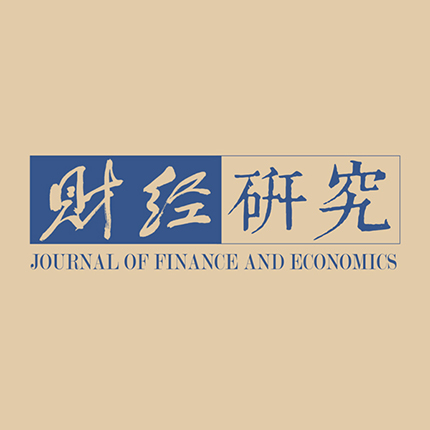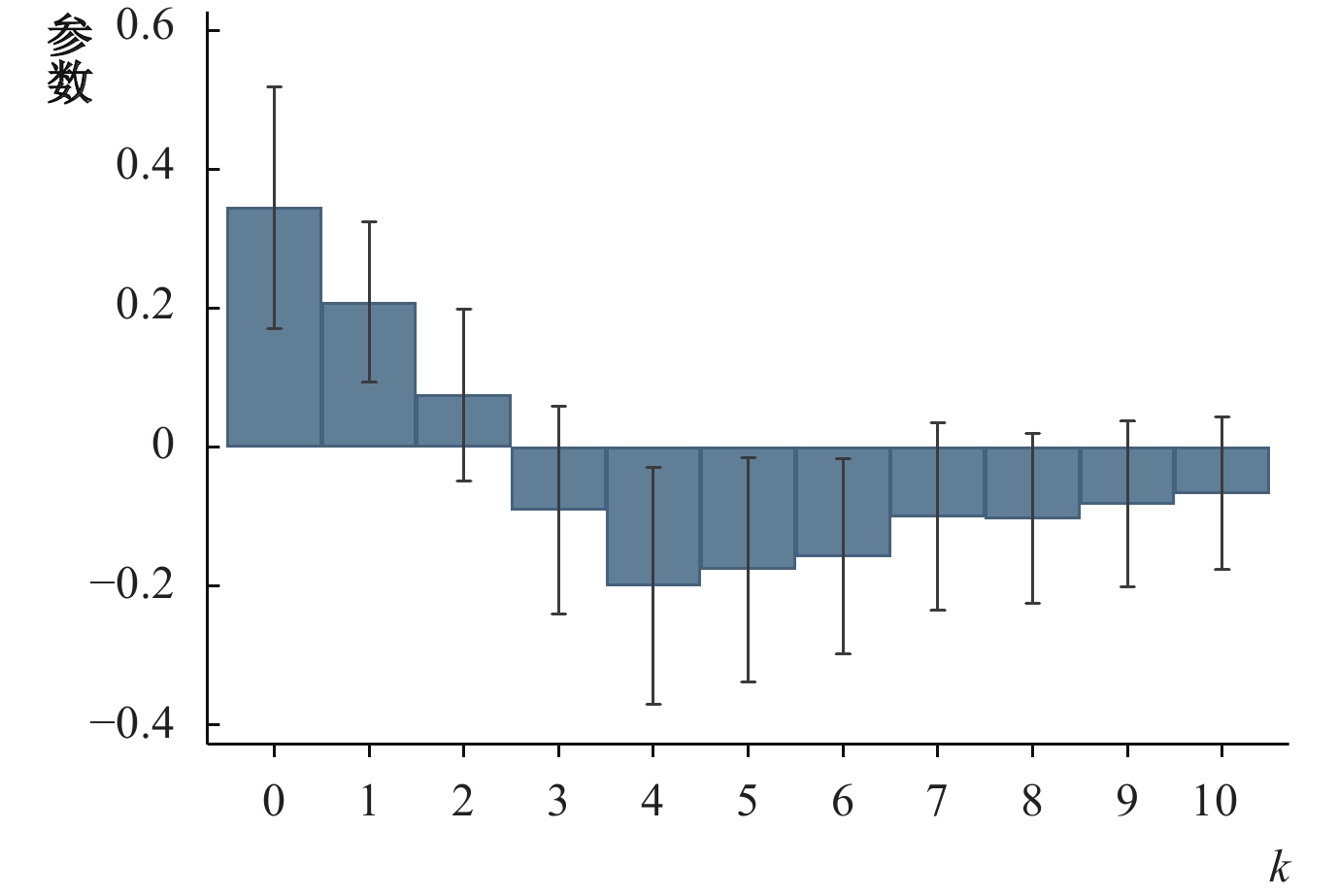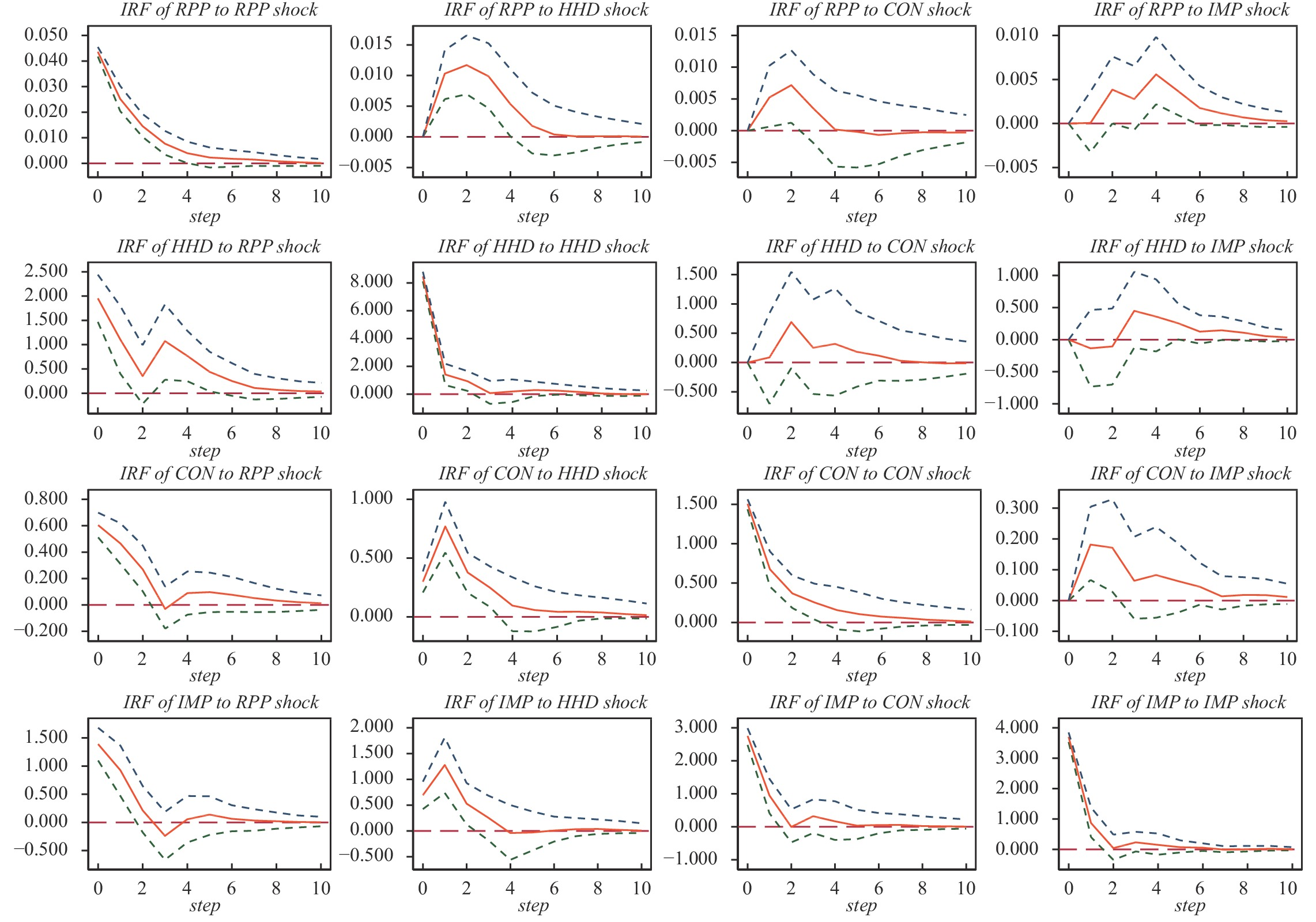﻿ 家庭债务周期、总需求与中国出口《财经研究》
2023第49卷第5期

1. 上海财经大学 高等研究院, 上海 200433;
2. 上海财经大学 经济学院, 上海 200433

Household Debt Cycles, Aggregate Demand and China’s Exports
Ning Lei1, Wang Ying2
1. Institute for Advanced Research, Shanghai University of Finance and Economics, Shanghai 200433, China;
2. School of Economics, Shanghai University of Finance and Economics, Shanghai 200433, China
Summary: Since the reform and opening up, China’s position in the world economy has been rising. Some countries have publicized the “China threat theory”. This paper offers a different explanation for China’s export growth. That is, the increase in total demand caused by the household debt expansion in importing countries is transmitted to international trade, which stimulates China’s exports. First of all, by constructing a theoretical model, this paper expounds the transmission path of household debt expansion to foreign trade, and clarifies the impact of household debt cycles on imports from China. In this two-phase model, households hold housing stock and housing loans in the first phase, and they sell the houses and pay off all the loans in the second phase. Based on this model, this paper proposes three hypotheses: (1) The increase of household debt will promote the rise of consumption, and then increase the consumption of foreign goods. (2) In the period of household debt expansion and relatively cheap prices of Chinese products, household debt has a greater impact on China’s exports. (3) In the period of economic downturn or household sector deleveraging, household debt has a greater impact on China’s exports. Secondly, using the data of transnational credit and China’s exports from 1979 to 2018, this paper empirically tests the impact of the household debt expansion in importing countries on China’s exports. The results show that, with other factors unchanged, an increase of 1 percentage point in foreign household debt will increase China’s exports by about 0.428 percentage points in the same period. This result is established before and after China’s accession to the WTO and the financial crisis. In addition, this paper also finds that although household debt expansion will boost China’s export growth in the short term, it is not conducive to China’s export growth in the long term, which is also consistent with the study of household debt cycles on economic growth (Mian et al., 2017). Finally, this paper discusses the channel and internal mechanism of the impact of foreign household debt on China’s export growth. Using the panel VAR model, it is found that both rising housing prices and falling interest rates will expand household debt, and the resulting increase in consumption will promote the country’s imports to other countries in the world, including China. The finding is consistent with the result estimated by the 2SLS method using housing prices and interest rates as household debt instrument variables. This paper has the following contributions: First, it provides a new explanation for China’s export growth. Second, it expands the research scope of consumption demand channel of household debt expansion, and points out that the total demand expansion caused by household debt cycles can spill over to other global economies. At the same time, this paper is also of great value to understand the importance of “dual circulation”.
Key words: household debt    China’s exports    “China Shock”

 $C={\left[\alpha {c}_{D}^{\rho }+\left(1-\alpha \right){c}_{F}^{\rho }\right]}^{1/\rho }$

 ${c}_{D}={\left(\frac{\alpha }{{p}_{D}}\right)}^{\sigma }\frac{C}{{\alpha }^{\sigma }{p}_{D}^{1-\sigma }+{\left(1-\alpha \right)}^{\sigma }{p}_{F}^{1-\sigma }}$
 ${c}_{F}={\left(\frac{1-\alpha }{{p}_{F}}\right)}^{\sigma }\frac{C}{{\alpha }^{\sigma }{p}_{D}^{1-\sigma }+{\left(1-\alpha \right)}^{\sigma }{p}_{F}^{1-\sigma }}$

 $\max\left[U\left({C}_{1}\right)+\beta U\left({C}_{2}\right)\right]$

 ${C}_{1}+r{M}_{0}+{A}_{1}={Y}_{1}+{M}_{1}+\left(1+r\right){A}_{0}$ (1)
 ${M}_{1}\le ph\times H-{M}_{0}$ (2)
 ${C}_{2}={Y}_{2}+ph\times H-\left(1+r\right)\left({M}_{0}+{M}_{1}\right)+{\left(1+r\right)A}_{1}$ (3)

1. 如果抵押约束是无约束力的（Non-Binding），也就是家庭的借贷没有超过最大限值，那么家庭第一期的最优消费表达式为：

 ${C}_{1}=\frac{1}{1+\beta }\left[{Y}_{1}+\frac{{Y}_{2}}{1+r}-\left(1+r\right){M}_{0}+\frac{ph\times H}{1+r}\right]$

 ${c}_{F}={\left(\frac{1-\alpha }{{p}_{y}}\right)}^{\sigma }\dfrac{\left[{Y}_{1}+\dfrac{{Y}_{2}}{1+r}-\left(1+r\right){M}_{0}+\dfrac{ph\times H}{1+r}\right]}{\left(1+\beta \right)\left({\alpha }^{\sigma }{p}_{x}^{1-\sigma }+{\left(1-\alpha \right)}^{\sigma }{p}_{y}^{1-\sigma }\right)}$

 ${M}_{1}=\frac{{Y}_{2}+ph\times H}{\left(1+\beta \right)\left(1+r\right)}-\frac{\beta }{1+\beta }{Y}_{1}-\frac{1-\beta r}{1+\beta }{M}_{0}$

2. 如果抵押约束是有约束力的（Binding），即家庭的借贷达到了最大值，此时家庭第一期的消费为：

 ${C}_{1}={Y}_{1}+ph\times H-\left(1+r\right){M}_{0}$

 ${c}_{F}={\left(\frac{1-\alpha }{{p}_{y}}\right)}^{\sigma }\frac{{Y}_{1}+ph\times H-\left(1+r\right){M}_{0}}{{\alpha }^{\sigma }{p}_{x}^{1-\sigma }+{\left(1-\alpha \right)}^{\sigma }{p}_{y}^{1-\sigma }}$

 ${M}_{1}=ph\times H-{M}_{0}$

H1：家庭债务的增加会推动消费的上升，进而增加对国外商品的消费，在本文中这种消费体现为对中国产品的需求。

H2：在家庭债务扩张且中国产品价格相对便宜的时期，家庭债务对中国出口的影响更大。

H3：在经济下行或者家庭部门降杠杆时，家庭债务对中国出口的影响更大。

（一）家庭债务扩张与中国出口增长

 ${\Delta }_{4}\mathrm{l}\mathrm{n}{EXP}_{it}={\beta }^{HH}{\Delta }_{4}\mathrm{l}\mathrm{n}{D}_{it}^{HH}+{\beta }^{F}{\Delta }_{4}{\mathrm{l}\mathrm{n}D}_{it}^{F}+{X}_{it}^{{\text{'}}}{\Gamma }+{u}_{i}+{\theta }_{t}+{\varepsilon}_{it}$ (4)

 （1） （2） （3） （4） （5） （6） ${\Delta }_{4}{\mathrm{l}\mathrm{n}D}_{it}^{HH}$ 0.302***（0.051） 0.346***（0.089） 0.259**（0.103） 0.428***（0.058） ${\Delta }_{4}{\mathrm{l}\mathrm{n}D}_{it}^{F}$ 0.297***（0.092） −0.092（0.141） −0.035（0.144） 0.076（0.112） ${\Delta }_{4}{\mathrm{l}\mathrm{n}D}_{it}^{Private}$ 0.352***（0.089） 控制变量 不控制 不控制 不控制 不控制 控制 控制 国家固定效应 控制 控制 控制 控制 控制 控制 年份固定效应 控制 控制 控制 控制 控制 控制 N 984 984 984 984 984 984 R2 0.635 0.610 0.619 0.636 0.639 0.796 注：标准误均为国家和年份层面的聚类标准误，*、**和***分别表示在10%、5%和1%的水平上显著。下同。

 （1）加入WTO前 （2）加入WTO后 （3）全样本 ${\Delta }_{4}{\mathrm{l}\mathrm{n}D}_{it}^{HH}$ 0.491***（0.153） 0.451***（0.094） 0.403***（0.088） ${\Delta }_{4}{\mathrm{l}\mathrm{n}D}_{it}^{F}$ −0.129（0.211） 0.047（0.128） 0.037（0.191） ${\Delta }_{4}{\mathrm{l}\mathrm{n}D}_{it}^{HH}\times 1\left(After2001\right)$ 0.021（0.107） ${\Delta }_{4}{\mathrm{l}\mathrm{n}D}_{it}^{F}\times 1\left(After2001\right)$ 0.068（0.176） 控制变量 控制 控制 控制 国家固定效应 控制 控制 控制 年份固定效应 控制 控制 控制 N 343 641 984 R2 0.752 0.883 0.796

（二）异质性分析

1. 家庭债务周期的非对称性。家庭债务主要通过家庭消费需求渠道对中国出口产生影响。对处于信贷扩张的国家来说，家庭债务扩张会使得家庭放松预算约束，增加中国出口；而在信贷紧缩的国家，家庭会收紧预算约束，进而对中国出口产生负向影响。表3列（1）仅考虑家庭债务扩张的样本，列（2）仅考虑家庭债务紧缩的样本，列（3）则构建了该国在 $t$ 期是否正经历家庭或企业债务加杠杆或降杠杆的虚拟变量，并将该虚拟变量分别与家庭或企业债务增速对应交互。如表3所示，家庭债务扩张对中国出口有正向促进作用，而家庭债务紧缩则会减少中国出口，同时家庭债务紧缩对中国出口的影响远大于家庭债务扩张。这也进一步说明中国出口主要受国外需求驱动，即当家庭部门加杠杆时，中国出口被用来填补进口国国内供给的不足；但当家庭部门降杠杆时，家庭部门可能更多地降低外部需求，减少对中国产品的消费。

 （1）信贷扩张 （2）信贷收缩 （3）全样本 ${\Delta }_{4}{\mathrm{l}\mathrm{n}D}_{it}^{HH}$ 0.336***（0.070） 0.547***（0.156） ${\Delta }_{4}{lnD}_{it}^{F}$ 0.023（0.088） 0.111（0.302） ${\Delta }_{4}{\mathrm{l}\mathrm{n}D}_{it}^{HH}\times 1({\Delta }_{4}{\mathrm{l}\mathrm{n}D}_{it}^{HH} > 0)$ 0.348***（0.057） ${\Delta }_{4}{\mathrm{l}\mathrm{n}D}_{it}^{HH}\times 1({\Delta }_{4}{\mathrm{l}\mathrm{n}D}_{it}^{HH}\leqslant 0)$ 0.561***（0.096） ${\Delta }_{4}{\mathrm{l}\mathrm{n}D}_{it}^{F}\times 1({\Delta }_{4}{\mathrm{l}\mathrm{n}D}_{it}^{F} > 0)$ 0.094（0.113） ${\Delta }_{4}{\mathrm{l}\mathrm{n}D}_{it}^{F}\times 1({\Delta }_{4}{\mathrm{l}\mathrm{n}D}_{it}^{F}\leqslant 0)$ 0.165（0.166） 控制变量 控制 控制 控制 国家固定效应 控制 控制 控制 年份固定效应 控制 控制 控制 N 763 218 984 R2 0.773 0.868 0.798

 （1）2003—2007年 （2）2008—2012年 （3）2013—2017年 ${\Delta }_{4}{\mathrm{l}\mathrm{n}D}_{it}^{HH}$ 0.359***（0.043） 0.700***（0.175） 0.257***（0.093） ${\Delta }_{4}{\mathrm{l}\mathrm{n}D}_{it}^{F}$ −0.047（0.114） −0.112（0.238） −0.067（0.103） 控制变量 控制 控制 控制 国家固定效应 控制 控制 控制 年份固定效应 控制 控制 控制 N 177 190 200 R2 0.805 0.617 0.740

2. 对中国产品的消费偏好程度。考虑到不同国家对中国产品的偏好度存在差异，即理论模型中不同国家的 $\alpha$ 不同，可能导致家庭债务扩张对中国产品的需求有差异。为此，本文使用中国加入WTO前中国对某国的出口值占该国总进口值的比重作为该国对中国产品偏好程度的代理变量，并据此将样本分为对中国产品的低偏好国家和高偏好国家。从表5可以看出，对中国产品偏好程度越高，该国的家庭债务扩张对中国出口的影响越大，这也说明各国对中国产品的偏好确实会放大债务周期对中国出口的影响。

 （1）低偏好国家 （2）高偏好国家 （3）全样本 ${\Delta }_{4}{\mathrm{l}\mathrm{n}D}_{it}^{HH}$ 0.278***（0.082） 0.484***（0.084） 0.325***（0.077） ${\Delta }_{4}{\mathrm{l}\mathrm{n}D}_{it}^{F}$ 0.144（0.134） 0.072（0.182） 0.207（0.144） ${\Delta }_{4}{\mathrm{l}\mathrm{n}D}_{it}^{HH}\times 1\left(Higher\right)$ 0.157**（0.075） ${\Delta }_{4}{\mathrm{l}\mathrm{n}D}_{it}^{F}\times 1\left(Higher\right)$ −0.226*（0.133） 控制变量 控制 控制 控制 国家固定效应 控制 控制 控制 年份固定效应 控制 控制 控制 N 544 440 984 R2 0.837 0.777 0.797

3. 经济发展程度和区域异质性。考虑到不同发展阶段的国家对外依存度不同，且各国间贸易可能受地域等因素的影响，本文从经济发展程度和区域位置两个方面进行了异质性分析。

 发达/发展中国家 高/低收入国家 （1）发达 （2）发展中 （3）全样本 （4）高收入 （5）低收入 （6）全样本 ${\Delta }_{4}{\mathrm{l}\mathrm{n}D}_{it}^{HH}$ 0.363***（0.079） 0.355***（0.063） 0.395***（0.080） 0.365***（0.061） 0.430***（0.067） 0.408***（0.112） ${\Delta }_{4}{\mathrm{l}\mathrm{n}D}_{it}^{F}$ −0.002（0.109） 0.046（0.108） 0.144（0.149） 0.137（0.118） −0.063（0.097） 0.140（0.207） ${\Delta }_{4}{\mathrm{l}\mathrm{n}D}_{it}^{HH}\times 1\left(Developed\right)$ 0.086（0.100） ${\Delta }_{4}{\mathrm{l}\mathrm{n}D}_{it}^{F}\times 1\left(Developed\right)$ −0.133（0.152） ${\Delta }_{4}{\mathrm{l}\mathrm{n}D}_{it}^{HH}\times 1\left(Highincome\right)$ 0.016（0.107） ${\Delta }_{4}{\mathrm{l}\mathrm{n}D}_{it}^{F}\times 1\left(Highincome\right)$ −0.079（0.197） 控制变量 控制 控制 控制 控制 控制 控制 国家固定效应 控制 控制 控制 控制 控制 控制 年份固定效应 控制 控制 控制 控制 控制 控制 N 715 269 984 801 183 984 R2 0.803 0.949 0.796 0.780 0.954 0.796

 （1）欧洲 （2）北美洲 （3）亚洲 ${\Delta }_{4}{\mathrm{l}\mathrm{n}D}_{it}^{HH}$ 0.339***（0.090） 0.841***（0.152） 0.433***（0.116） ${\Delta }_{4}{\mathrm{l}\mathrm{n}D}_{it}^{F}$ 0.025（0.161） −0.970***（0.263） −0.087（0.221） 控制变量 控制 控制 控制 国家固定效应 控制 控制 控制 年份固定效应 控制 控制 控制 N 557 72 200 R2 0.793 0.977 0.886

（三）稳健性检验

1. 内生性处理。在基准回归中，本文采用长差分固定效应模型能控制住未被观测到的国家异质效应。但是，国外家庭债务与中国出口间可能存在联立性偏误，因为国外家庭债务扩张会促进中国出口，而中国出口使得国外市场竞争加剧，导致家庭收入等受到影响，家庭被动增加债务，这也是“中国冲击论”所持的观点。为此，本文通过使用房价增速和同期政策利率作为家庭债务变动的工具变量，结果发现基准模型低估了家庭债务扩张对中国出口增长的促进作用。同时，由于本文数据横跨四十年，各国宏观变量可能存在序列相关性，因此本文通过加入因变量的一阶滞后项建立动态面板模型，采用差分GMM和系统GMM进行检验，结果发现基准回归结果稳健。

2. 差分间隔。本文已经检验了将长差分时间间隔设置为四年的情形。为避免时间间隔选取的特殊性会影响估计结果，本文分别检验了一年、两年、三年和五年时间间隔的长差分，结果发现系数都在1%的水平上显著为正。

3. 样本选取方式。本文共考虑以下三种样本选取方式：（1）将样本拓展到1955—2019年；（2）在变量构造时，仅采用非重叠的四年间隔样本，即 $\left\{{\Delta }_{4}{y}_{t},{\Delta }_{4}{y}_{t-1},{\Delta }_{4}{y}_{t-2},{\Delta }_{4}{y}_{t-3},{\Delta }_{4}{y}_{t-4},{\Delta }_{4}{y}_{t-5}\right\}$ 仅选用 $\left\{{\Delta }_{4}{y}_{t},{\Delta }_{4}{y}_{t-5}\right\}$ ；（3）考察欧盟和OECD等经济贸易组织样本中该效应的稳健性。结果均表明家庭债务扩张对中国出口增长的促进作用是显著的。

4. 不同固定效应情形。考虑到各国不随时间变动的异质性以及经济增长等因素，本文在基准模型中加入了国家固定效应和时间固定效应。这里又考察了以下情形：（1）不控制国家固定效应和时间固定效应；（2）仅控制国家固定效应；（3）仅控制时间固定效应；（4）控制国家固定效应和线性时间趋势。结果发现，虽然不同情形下系数大小略有差异，但系数均在1%的水平上显著。

5. 国内外经济因素。本文从各国国内经济环境和中国国内经济环境两个方面考察基准回归结果的稳健性。首先，从各国国内经济环境考虑如下因素：（1）经济增速；（2）政府债务变动；（3）国家主权债务；（4）核心解释变量替换为杠杆率变动。其次，从中国国内经济环境考虑如下因素：（1）产业结构；（2）研发投入；（3）企业信息化程度；（4）企业互联网交易程度。结果表明，国外家庭债务扩张对中国出口的促进作用是显著的。

（四）家庭债务周期的中长期影响

 ${\Delta }_{4}\mathrm{l}\mathrm{n}{EXP}_{it+k}={\beta }_{k}^{HH}{\Delta }_{4}\mathrm{l}\mathrm{n}{D}_{it}^{HH}+{\beta }_{k}^{F}{\Delta }_{4}{\mathrm{l}\mathrm{n}D}_{it}^{F}+{u}_{i}+{\theta }_{t}+{\varepsilon}_{it}\quad\quad k={0,1},\cdots, 10$ (5)图 1 家庭债务扩张与中国出口增长的动态变化

 （1）家庭消费 （2）总消费 （3）总进口 （4）进口（不含中国） ${\Delta }_{4}{\mathrm{l}\mathrm{n}D}_{it}^{HH}$ 0.099***（0.018） 0.094***（0.013） 0.135**（0.057） 0.172***（0.045） ${\Delta }_{4}{\mathrm{l}\mathrm{n}D}_{it}^{F}$ 0.053*（0.031） 0.047*（0.028） 0.081（0.070） 0.082（0.057） 控制变量 控制 控制 控制 控制 国家固定效应 控制 控制 控制 控制 年份固定效应 控制 控制 控制 控制 N 992 944 970 970 R2 0.582 0.599 0.580 0.693图 2 中央银行政策利率、家庭债务、家庭消费和总进口的四变量脉冲效应图图 3 实际住宅价格、家庭债务、家庭消费和总进口的四变量脉冲效应图

① 本文的基准实证分析均采用1979—2018年全球40个国家的非平衡面板数据。受篇幅限制，文中未报告数据和描述性统计；如有需要，可向作者索取。

② 本文借鉴Mian等（2017）的VAR方法，发现样本信贷扩张区间是四年。受篇幅限制，文中对此未予以报告；如有需要，可向作者索取。

③ 受篇幅限制，文中未报告政府债务增长的回归结果；如有需要，可向作者索取。

④ 感谢匿名审稿人提出的宝贵建议。工作论文中的附录1表9A采用DID方法分析了国际金融危机这一冲击的影响。

⑤ 受篇幅限制，文中未报告稳健性检验结果；如有需要，可向作者索取。

⑥ 受篇幅限制，文中未报告家庭杠杆率与家庭消费和总进口的散点图；如有需要，可向作者索取。

⑦ 对所有的指标进行IPS和费雪检验的结果均表明，除中央银行政策利率外，其余所有的变量都是一阶单整的非平稳变量，经对数差分处理后均为平稳性变量，可以纳入面板VAR模型考察它们之间的关系。

⑧ 如金融时报的评论就指出，疫情后中国持续增加的出口可能会对中国经济的再平衡产生影响。

  戴美虹, 刘海洋, 林令涛. 制度还是技术影响中国的出口活力: 以国有企业为例[J]. 世界经济, 2021(3): 110–134.  纪洋, 葛婷婷, 边文龙, 等. 杠杆增速、部门差异与金融危机——“结构性去杠杆”的实证分析与我国杠杆政策的讨论[J]. 经济学(季刊), 2021(3): 843–862. DOI:10.13821/j.cnki.ceq.2021.03.05  李兵, 李柔. 互联网与企业出口: 来自中国工业企业的微观经验证据[J]. 世界经济, 2017(7): 102–125.  刘志彪, 张杰. 我国本土制造业企业出口决定因素的实证分析[J]. 经济研究, 2009(8): 99–112.  鲁晓东, 刘京军. 不确定性与中国出口增长[J]. 经济研究, 2017(9): 39–54.  马述忠, 张洪胜. 集群商业信用与企业出口——对中国出口扩张奇迹的一种解释[J]. 经济研究, 2017(1): 13–27.  马勇, 陈雨露. 金融杠杆、杠杆波动与经济增长[J]. 经济研究, 2017(6): 31–45.  毛其淋, 盛斌. 贸易自由化、企业异质性与出口动态——来自中国微观企业数据的证据[J]. 管理世界, 2013(3): 48–68.  施炳展. 互联网与国际贸易——基于双边双向网址链接数据的经验分析[J]. 经济研究, 2016(5): 172–187.  王海成, 许和连, 邵小快. 国有企业改制是否会提升出口产品质量[J]. 世界经济, 2019(3): 94–117. DOI:10.19985/j.cnki.cassjwe.2019.03.006  许和连, 王海成. 简政放权改革会改善企业出口绩效吗?——基于出口退(免)税审批权下放的准自然试验[J]. 经济研究, 2018(3): 157–170.  Asquith B, Goswami S, Neumark D, et al. U. S. job flows and the China shock[J]. Journal of International Economics, 2019, 118: 123–137. DOI:10.1016/j.jinteco.2019.02.002  Autor D, Dorn D, Hanson G H. When work disappears: Manufacturing decline and the falling marriage market value of young men[J]. American Economic Review:Insights, 2019, 1(2): 161–178. DOI:10.1257/aeri.20180010  Autor D, Dorn D, Hanson G H, et al. Importing political polarization? The electoral consequences of rising trade exposure[J]. American Economic Review, 2020, a,110(10): 3139–3183.  Autor D, Dorn D, Hanson G H, et al. Foreign competition and domestic innovation: Evidence from US patents[J]. American Economic Review:Insights, 2020, b,2(3): 357–374.  Autor D H, Dorn D, Hanson G H. The China syndrome: Local labor market effects of import competition in the United States[J]. American Economic Review, 2013, 103(6): 2121–2168. DOI:10.1257/aer.103.6.2121  Autor D H, Dorn D, Hanson G H, et al. Trade adjustment: Worker-level evidence[J]. The Quarterly Journal of Economics, 2014, 129(4): 1799–1860. DOI:10.1093/qje/qju026  Bernard A B, Fort T C, Smeets V, et al. Heterogeneous globalization: Offshoring and reorganization[R]. NBER Working Paper No. 26854, 2020.  Bhutta N, Keys B J. Interest rates and equity extraction during the housing boom[J]. American Economic Review, 2016, 106(7): 1742–1774. DOI:10.1257/aer.20140040  Kaplan G, Violante G L. A model of the consumption response to fiscal stimulus payments[J]. Econometrica, 2014, 82(4): 1199–1239. DOI:10.3982/ECTA10528  Mian A, Sufi A. House prices, home equity-based borrowing, and the US household leverage crisis[J]. American Economic Review, 2011, 101(5): 2132–2156. DOI:10.1257/aer.101.5.2132  Mian A, Sufi A. Household debt and defaults from 2000 to 2010: The credit supply view[A]. Fennell L A, Keys B J. Evidence and innovation in housing law and policy[C]. Cambridge: Cambridge University Press, 2017, 257-288.  Mian A, Sufi A. Finance and business cycles: The credit-driven household demand channel[J]. Journal of Economic Perspectives, 2018, 32(3): 31–58. DOI:10.1257/jep.32.3.31  Mian A, Sufi A, Verner E. Household debt and business cycles worldwide[J]. The Quarterly Journal of Economics, 2017, 132(4): 1755–1817. DOI:10.1093/qje/qjx017  Mian A, Sufi A, Verner E. How does credit supply expansion affect the real economy? The productive capacity and household demand channels[J]. The Journal of Finance, 2020, a,75(2): 949–994.  Mian A, Straub L, Sufi A. Indebted demand[R]. NBER Working Paper No. 26940, 2020b.  Paz L S. The effect of import competition on Brazil’s manufacturing labor market in the 2000s: Are imports from China different?[J]. The International Trade Journal, 2018, 32(1): 76–99. DOI:10.1080/08853908.2017.1389323  Pierce J R, Schott P K. The surprisingly swift decline of US manufacturing employment[J]. American Economic Review, 2016, 106(7): 1632–1662. DOI:10.1257/aer.20131578  Zeldes S P. Consumption and liquidity constraints: An empirical investigation[J]. Journal of Political Economy, 1989, 97(2): 305–346. DOI:10.1086/261605  Zhu B, Li L X, Downs D H, et al. New evidence on housing wealth and consumption channels[J]. The Journal of Real Estate Finance and Economics, 2019, 58(1): 51–79. DOI:10.1007/s11146-017-9638-8